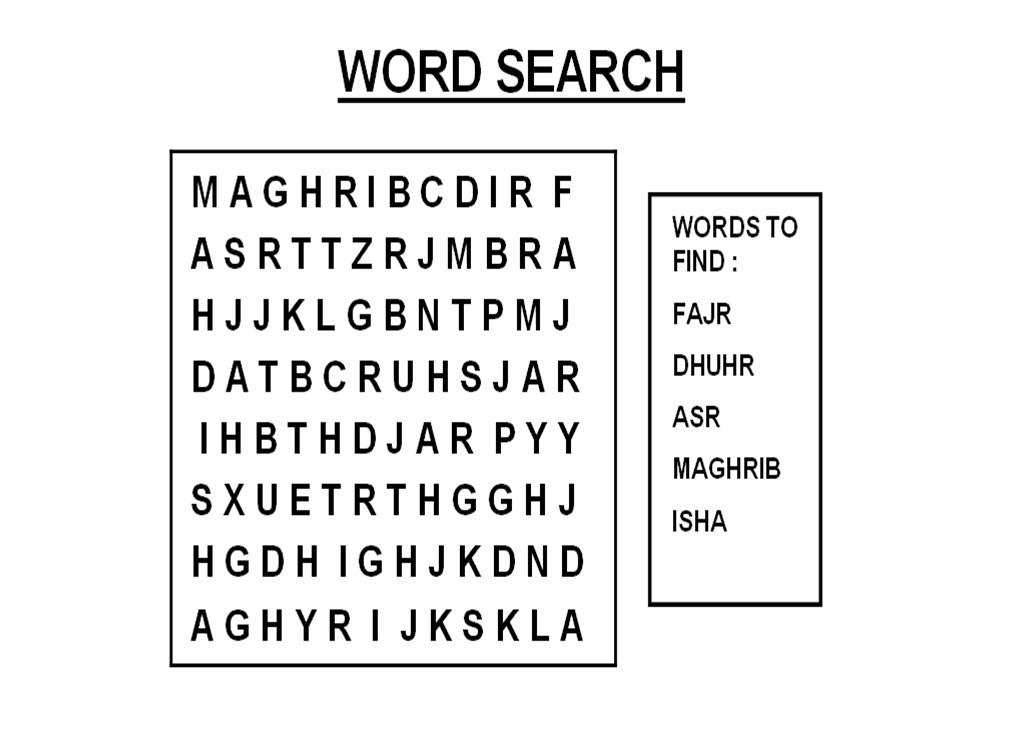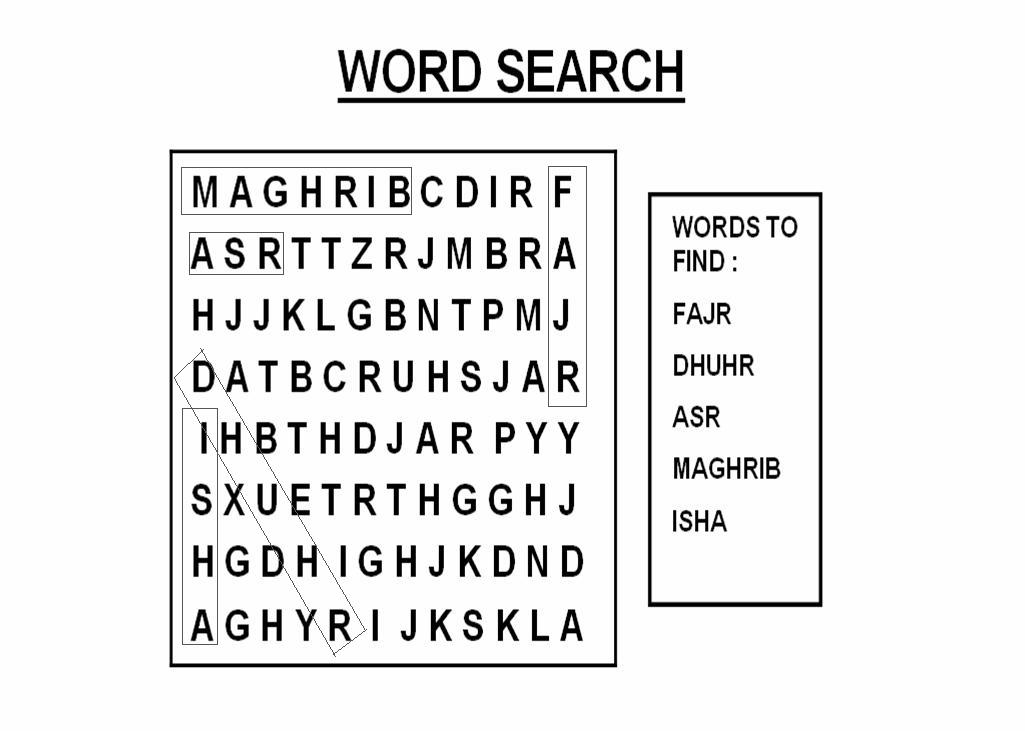# Ibtesam

Print this Word Search for the kids to enjoy and then share the answers with them. To print this Word Search, right-click on the image and save it on your computer to print.

Enjoy!W  T  R  R  V  O  W  O  V  L  F  G  V  F  A

R  I  X  K  Y  R  S  L  A  X  S  R  V  R  U

V  A  K  T  U  A  R  A  X  H  M  A  A  A  D

P  J  F  I  M  B  D  W  R  L  U  M  J  B  A

H  U  H  A  V  I  H  W  G  A  H  A  J  I  V

X  M  N  U  S  U  I  A  C  W  A  D  I  U  S

N  A  P  Q  E  T  L  L  A  W  R  A  H  L  N

I  D  V  V  V  H  Q  U  D  A  R  N  L  A  U

Y  I  T  C  K  A  '  I  B  H  A  H  I  W  Z

M  U  U  Q  W  N  A  D  U  S  M  R  H  W  E

R  T  F  J  Q  I  D  A  R  Q  Q  A  D  A  L

N  H  U  U  F  M  A  M  Y  P  P  J  E  L  F

U  A  Y  F  Q  X  Q  U  B  E  P  A  X  X  W

N  N  O  F  X  H  K  J  O  S  K  B  U  Y  R

P  I  X  J  A  S  H  A  '  B  A  N  V  K  Y

 Muharram Safar Rabi ul Awwal Rabi ul thani Jumadi ul Awwal Jumadi uthani Rajab Sha'ban Ramadan Shawwal Dhil Q'ada Dhil Hijja

Place these islamic months in the correct  order in the given place below:

 Dhil Hijja Rabi uthani Dhil Q'ada Rajab Jumadi ul Awwal Ramadan Jumadi uthani Safar Muharram Sha'ban Rabiul Awwal Shawwal